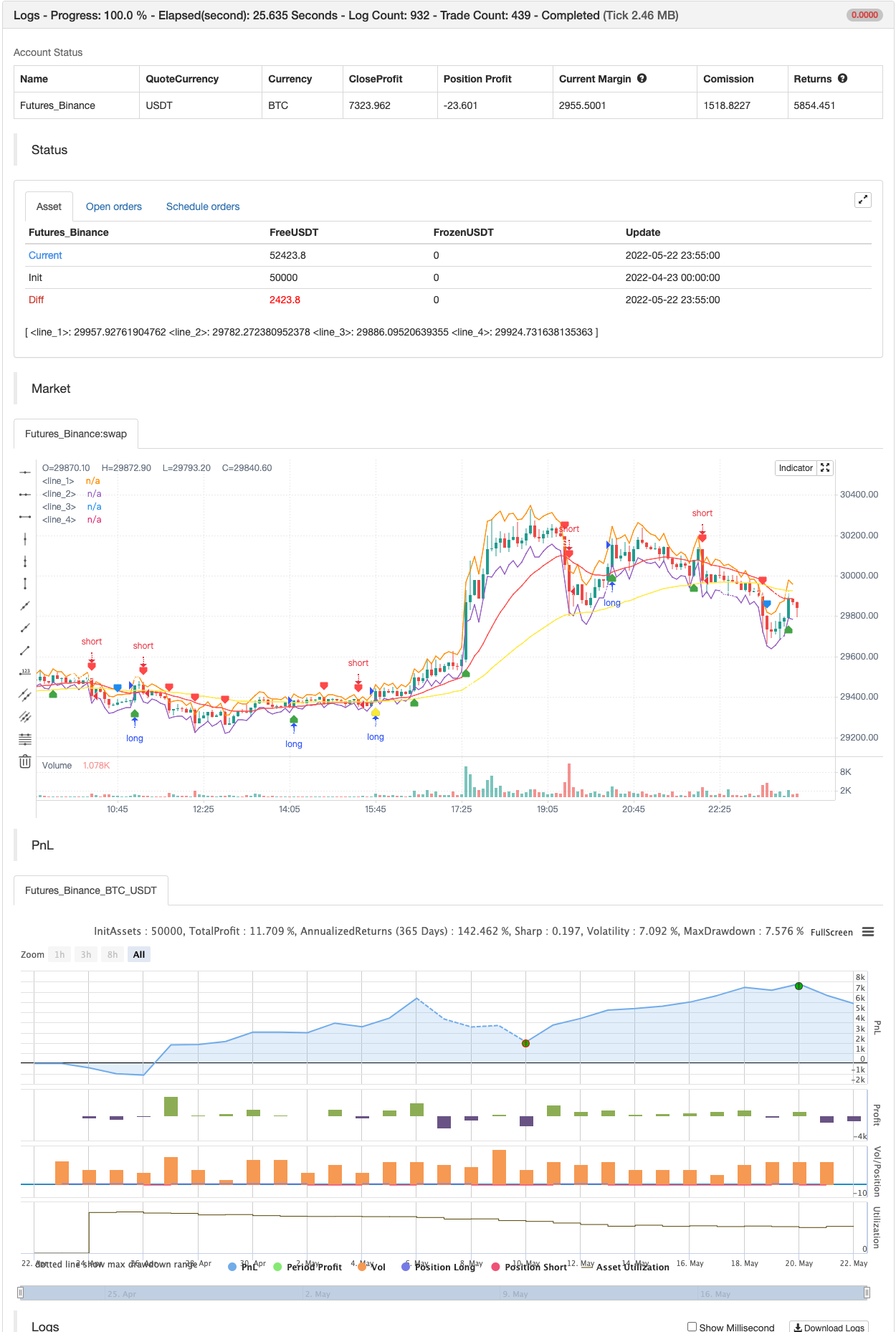# Super Scalper - 5 Min 15 Min

Author: 张超, Date: 2022-05-24 16:20:58
Tags: RMA sma EMA WMA RSI ATR

This strategy is based on RSI and ATR Bands which works better in 5 and 15 Mins time frame. Perform enough back testing with 1:2R before using in real time.

I have also added Golden Cross Over of 65 and 21 EMA to have confirmation on trend.

backtest```/*backtest
start: 2022-04-23 00:00:00
end: 2022-05-22 23:59:00
period: 5m
basePeriod: 1m
exchanges: [{"eid":"Futures_Binance","currency":"BTC_USDT"}]
*/

//@version=5
//Revision: Updated script to pine script version 5
//added Double RSI for Long/Short prosition trend confirmation instead of single RSI
strategy("Super Scalper - 5 Min 15 Min", overlay=true)
source = close
atrlen = input.int(14, "ATR Period")
mult = input.float(1, "ATR Multi", step=0.1)
smoothing = input.string(title="ATR Smoothing", defval="WMA", options=["RMA", "SMA", "EMA", "WMA"])
ma_function(source, atrlen) =>
if smoothing == "RMA"
ta.rma(source, atrlen)
else
if smoothing == "SMA"
ta.sma(source, atrlen)
else
if smoothing == "EMA"
ta.ema(source, atrlen)
else
ta.wma(source, atrlen)
atr_slen = ma_function(ta.tr(true), atrlen)
upper_band = atr_slen * mult + close
lower_band = close - atr_slen * mult

// Create Indicator's
ShortEMAlen = input.int(21, "Fast EMA")
LongEMAlen = input.int(65, "Slow EMA")
shortSMA = ta.ema(close, ShortEMAlen)
longSMA = ta.ema(close, LongEMAlen)
RSILen1 = input.int(25, "Fast RSI Length")
RSILen2 = input.int(100, "Slow RSI Length")
rsi1 = ta.rsi(close, RSILen1)
rsi2 = ta.rsi(close, RSILen2)
atr = ta.atr(atrlen)

//RSI Cross condition
RSILong = rsi1 > rsi2
RSIShort = rsi1 < rsi2

// Specify  conditions
longCondition = open < lower_band
shortCondition = open > upper_band
GoldenLong = ta.crossover(shortSMA,longSMA)
Goldenshort = ta.crossover(longSMA,shortSMA)

plotshape(shortCondition, title="Sell Label", text="Sell", location=location.abovebar, style=shape.labeldown, size=size.tiny, color=color.red, textcolor=color.white, transp=0)
plotshape(Goldenshort, title="Golden Sell Label", text="Golden Crossover Short", location=location.abovebar, style=shape.labeldown, size=size.tiny, color=color.blue, textcolor=color.white, transp=0)
plotshape(GoldenLong, title="Golden Buy Label", text="Golden Crossover Long", location=location.belowbar, style=shape.labelup, size=size.tiny, color=color.yellow, textcolor=color.white, transp=0)
// Execute trade if condition is True
if (longCondition)
stopLoss = low - atr * 2
takeProfit = high + atr * 5
strategy.entry("long", strategy.long, when = RSILong)

if (shortCondition)
stopLoss = high + atr * 2
takeProfit = low - atr * 5
strategy.entry("short", strategy.short, when = RSIShort)

// Plot ATR bands to chart

////ATR Up/Low Bands

plot(upper_band)
plot(lower_band)

// Plot Moving Averages
plot(shortSMA, color = color.red)
plot(longSMA, color = color.yellow)
```

Related

More